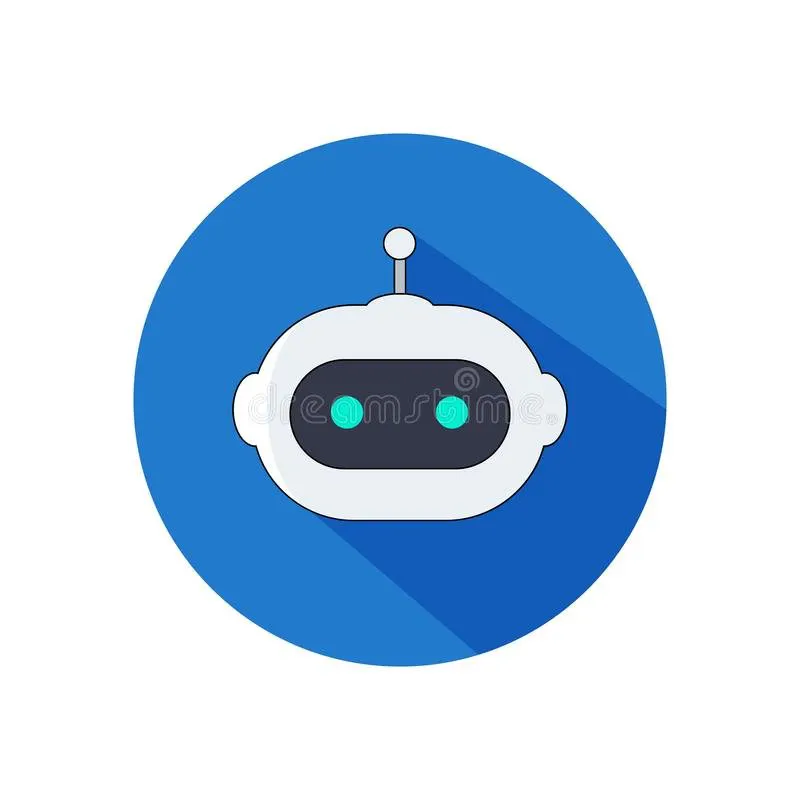# Temperature Sensor for Arduino applied for COVID 19

You'll learn how to construct a datalogger and monitor the temperature of patients with COVID 19

IntermediateFull instructions provided2 hours1,392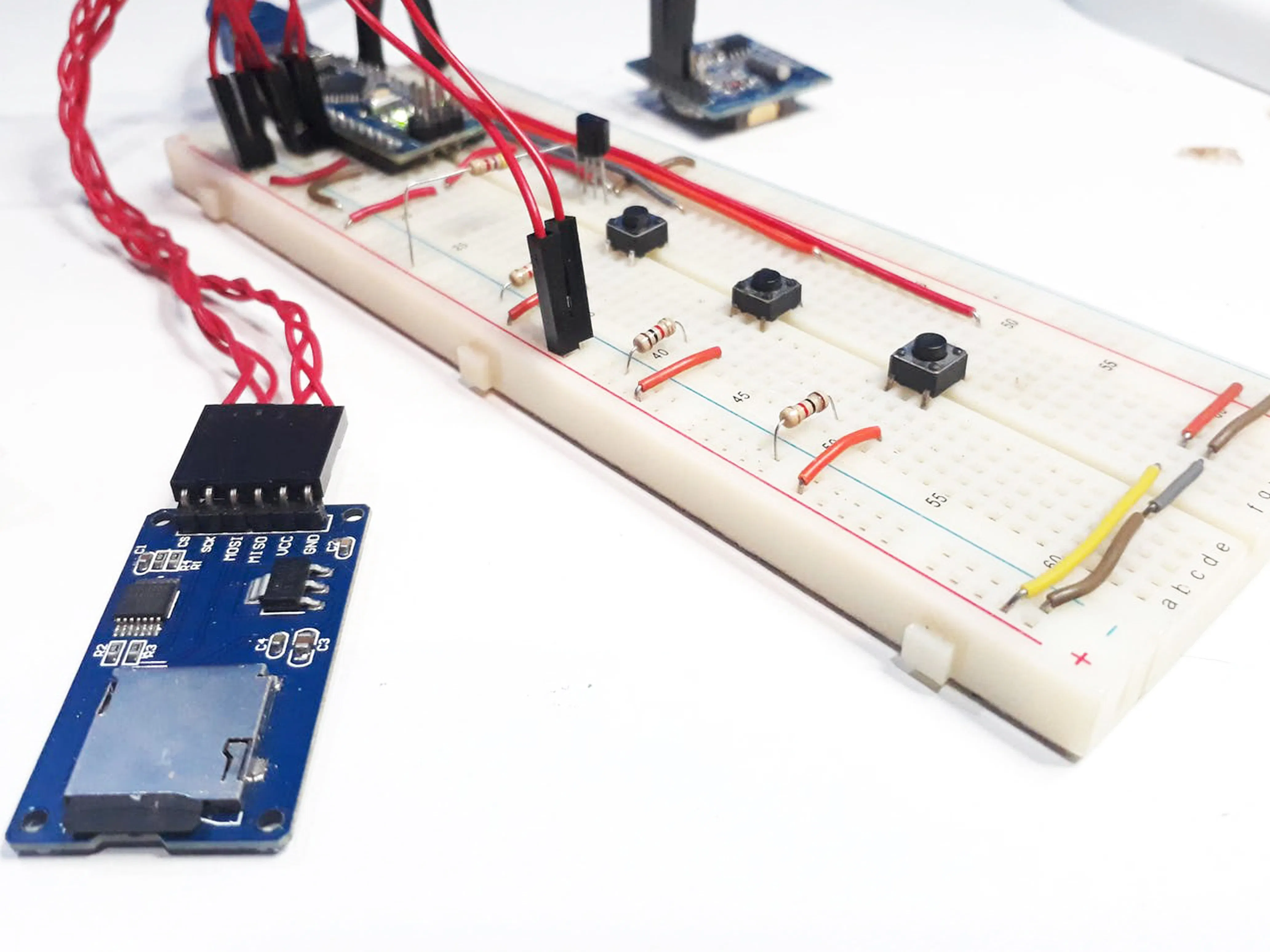## Things used in this project

### Hardware components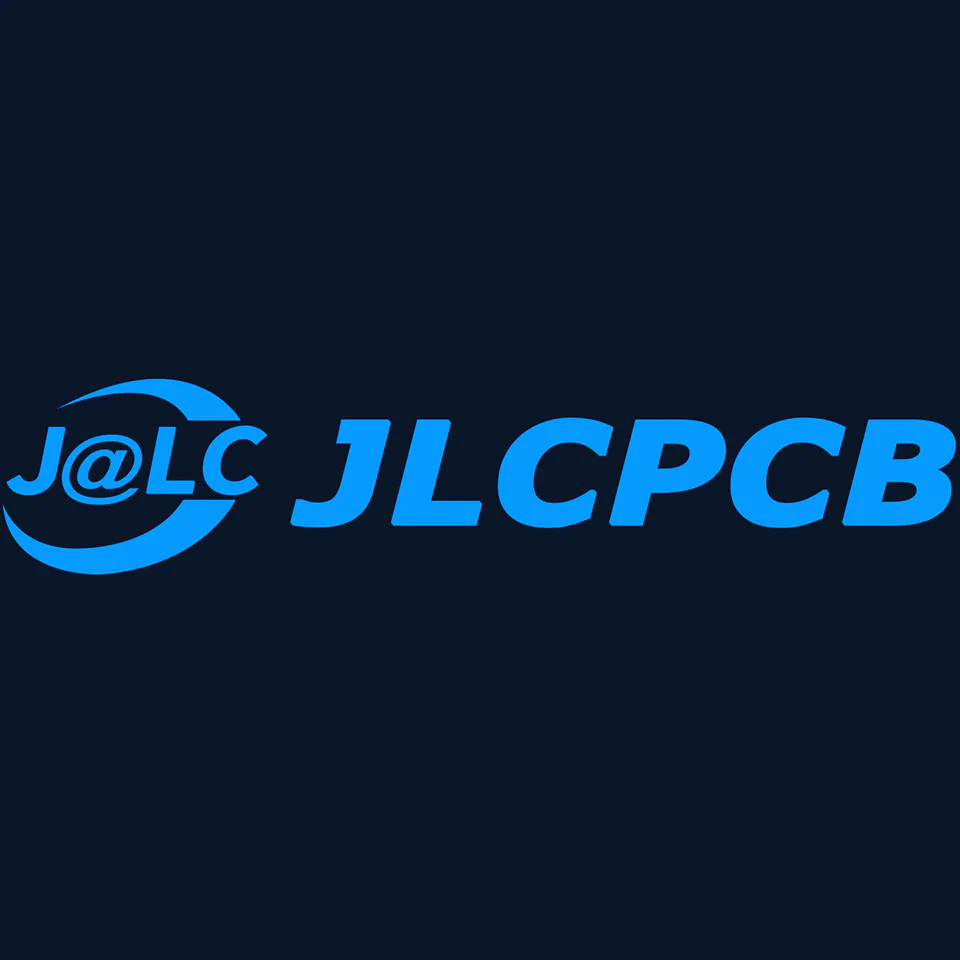JLCPCB Customized PCB
×1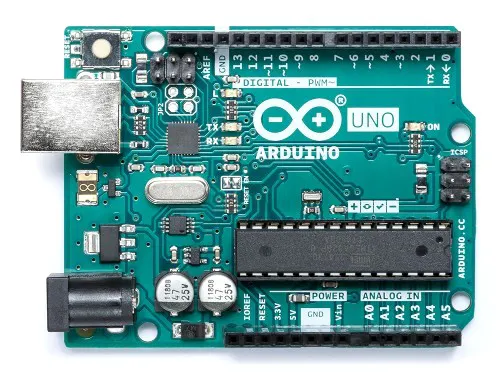JLCPCB Arduino Uno
×1
 JLCPCB Printed Circuit Board - Project
×1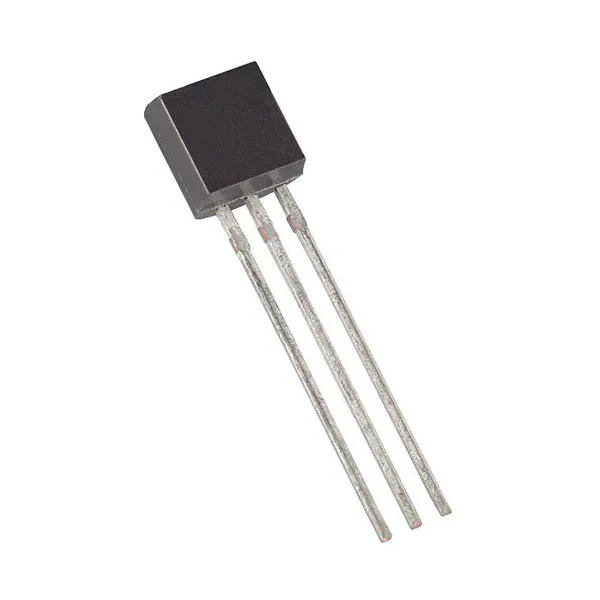Maxim Integrated DS18B20 Programmable Resolution 1-Wire Digital Thermometer
×1Arduino Nano R3
×1Jumper wires (generic)
×1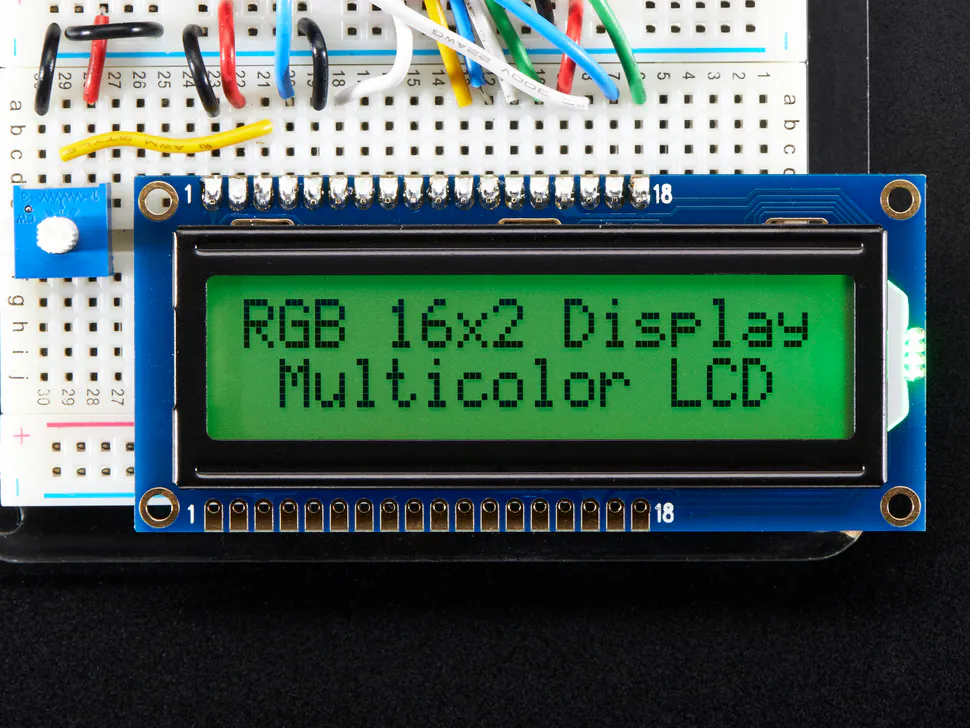Adafruit RGB Backlight LCD - 16x2
×1SparkFun Pushbutton switch 12mm
×1Resistor 1k ohm
×1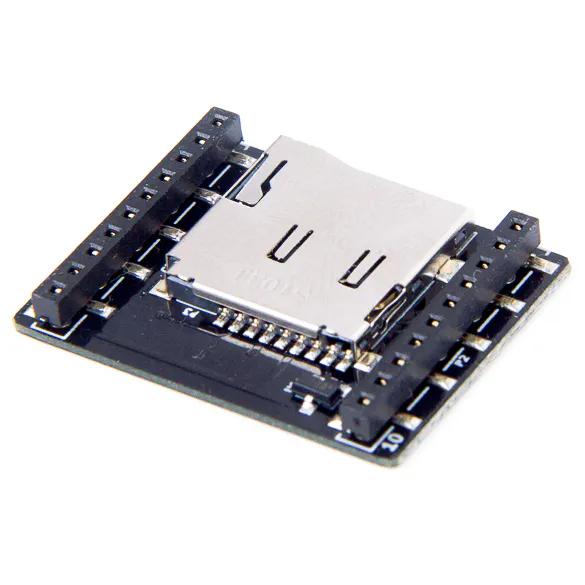Bitcraze Micro SD card deck
×1×1

### Software apps and online servicesArduino IDE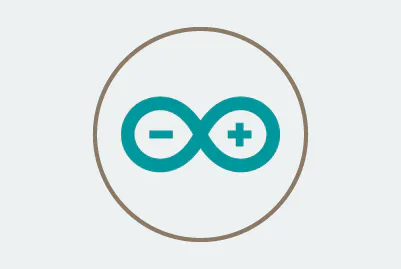Arduino Web Editor

## Schematics

### Datalogger with Temperature Sensor with Arduino - Schematic Electronic Project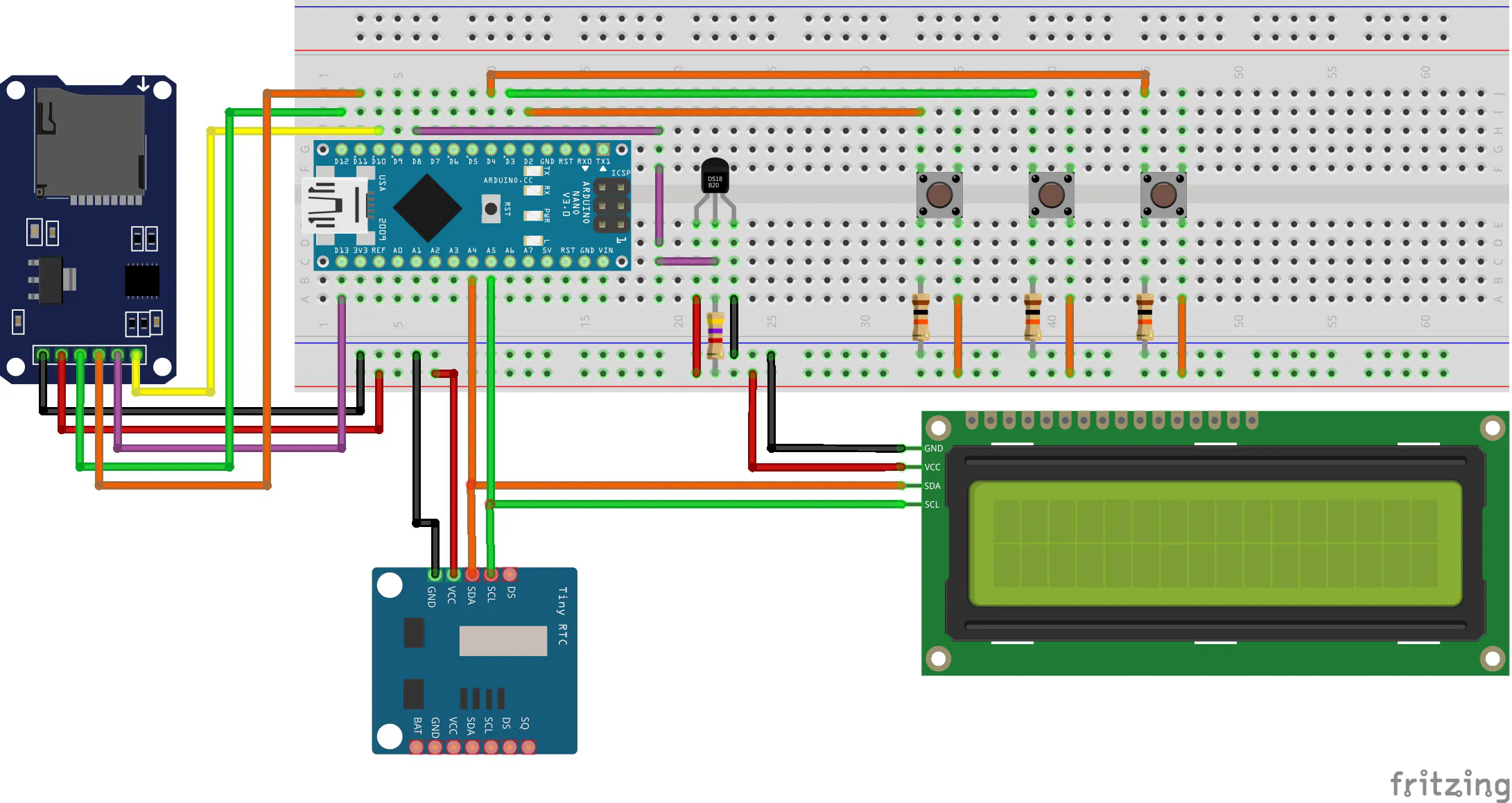## Code

### Datalogger with Temperature Sensor with Arduino - Code

Arduino
```#include <DallasTemperature.h> //Library with all function of DS18B20 Sensor
#include <DS1307.h>
#include <LiquidCrystal_I2C.h> //Biblioteca I2C do LCD 16x2
#include <Wire.h> //Biblioteca de Comunicacao I2C
#include <OneWire.h> //OneWire Library for DS18B20 Sensor
#include <SD.h>
#include <SPI.h>

LiquidCrystal_I2C lcd(0x27,16,2);  // Configurando o endereco do LCD 16x2 para 0x27

#define ONE_WIRE_BUS 8 //Digital Pin to connect the DS18B20 Sensor

//Define uma instancia do oneWire para comunicacao com o sensor
OneWire oneWire(ONE_WIRE_BUS);

DallasTemperature sensors(&oneWire);

File myFile;

#define Buttonmeasure 2
#define Buttonok 4

bool measure = 0, adjusthour = 0, ok = 0;
bool measure_state = 0, adjusthour_state = 0, ok_state = 0;
bool measure_process = 0, adjust_process = 0;

byte actualMin = 0, previousMin = 0;
byte actualHour = 0, previousHour = 0;
byte minUpdate = 0;

int pinoSS = 10; // Pin 53 para Mega / Pin 10 para UNO

char times = "";
int DataTime;

void updateHour()
{
DS1307.getDate(DataTime);

if(DataTime != minUpdate)
{
sprintf(times, "%02d:%02d", DataTime, DataTime);

lcd.setCursor(0,0);
lcd.print("                ");
lcd.setCursor(5,0);
lcd.print(times);

minUpdate = DataTime;
}

}

void updateTemp()
{
DS1307.getDate(DataTime);

if(DataTime != minUpdate)
{
sprintf(times, "%02d:%02d", DataTime, DataTime);

lcd.clear();
lcd.setCursor(5,0);
lcd.print(times);

lcd.setCursor(0,1);
lcd.print("Temperature: ");
lcd.setCursor(14,1);

sensors.requestTemperatures();
float TempSensor = 29;

lcd.print(TempSensor);

minUpdate = DataTime;
}

}

void setup()
{

Serial.begin(9600);
DS1307.begin();
sensors.begin();
pinMode(pinoSS, OUTPUT); // Declara pinoSS como saída

Wire.begin(); //Inicializacao da Comunicacao I2C
lcd.init();                      //Inicializacao do LCD
lcd.backlight();

lcd.setCursor(3,0);
lcd.print("Temp System");
lcd.setCursor(3,1);
lcd.print("Datalogger");
delay(2000);

// Localiza e mostra enderecos dos sensores
Serial.println("Localizando sensores DS18B20...");
Serial.print("Sensor Localization successfully!");
Serial.print(sensors.getDeviceCount(), DEC);
Serial.println(" Sensor");

if(SD.begin())
{
// Inicializa o SD Card
Serial.println("SD Card pronto para uso."); // Imprime na tela
}

else

{
Serial.println("Falha na inicialização do SD Card.");
return;
}

DS1307.getDate(DataTime);

lcd.clear();

sprintf(times, "%02d:%02d", DataTime, DataTime);

lcd.setCursor(5,0);
lcd.print(times);
lcd.setCursor(0,1);
lcd.print("1-M   2-H  3-O/P");
}

void loop()
{
updateHour();

if(measure == 0 && measure_state == 1)
{
measure_state = 0;
}

if(measure == 1 && measure_state == 0 && measure_process == 0)
{
measure_process = 1;
measure_state = 1;

if (SD.exists("temp.txt"))
{
Serial.println("Apagou o arquivo anterior!");
SD.remove("temp.txt");
myFile = SD.open("temp.txt", FILE_WRITE); // Cria / Abre arquivo .txt
Serial.println("Criou o arquivo!");
}

else

{
Serial.println("Criou o arquivo!");
myFile = SD.open("temp.txt", FILE_WRITE); // Cria / Abre arquivo .txt
myFile.close();
}

delay(500);

myFile.print("Hour: ");
myFile.println("Temperature");

DS1307.getDate(DataTime);
actualMin = previousMin = DataTime;

sprintf(times, "%02d:%02d", DataTime, DataTime);

lcd.clear();
lcd.setCursor(5,0);
lcd.print(times);

lcd.setCursor(0,1);
lcd.print("Temperature: ");
lcd.setCursor(14,1);

sensors.requestTemperatures();
float TempSensor = 29;

lcd.print(TempSensor);
}

{
}

{
}

//-------------------------------------------------------Measuring Process-----------------------------------------------------------
if(measure_process == 1)
{
updateTemp();

byte contMin = 0, contHour = 0;
DS1307.getDate(DataTime);
actualMin = DataTime;

//---------------------------------------------------------Count Minutes----------------------------------------------------------
if(actualMin != previousMin)
{
contMin++;
previousMin = actualMin;
}

if(contMin == 1)
{
sprintf(times, "%02d:%02d ", DataTime, DataTime);

sensors.requestTemperatures();
float TempSensor = 29;

myFile.print(times);
myFile.println(TempSensor);
contMin = 0;
}
//-----------------------------------------------------------Count Hours----------------------------------------------------------
if(actualHour != previousHour)
{
contHour++;
previousHour = actualHour;
}

if(contHour == 5)
{
myFile.close();
lcd.clear();
lcd.setCursor(5,0);
lcd.print("Finished");
lcd.setCursor(5,1);
lcd.print("Process");
measure_process = 0;
contHour = 0;
}
//----------------------------------------------Condition to stop the datalogger--------------------------------------------------
if(ok == 1)
{
myFile.close();

lcd.clear();
lcd.setCursor(6,0);
lcd.print("Stoped");
lcd.setCursor(5,1);
lcd.print("Process");

measure_process = 0;
delay(2000);

lcd.clear();

DS1307.getDate(DataTime);

sprintf(times, "%02d:%02d", DataTime, DataTime);

lcd.setCursor(5,0);
lcd.print(times);
lcd.setCursor(0,1);
lcd.print("1-M   2-H  3-O/P");
}

}

{
lcd.clear();
DS1307.getDate(DataTime);
lcd.setCursor(0,0);

sprintf(times, "%02d:%02d", DataTime, DataTime);

lcd.setCursor(5,1);
lcd.print(times);

do
{

if(measure == 0 && measure_state == 1)
{
measure_state = 0;
}

if(measure == 1 && measure_state == 0)
{
DataTime++;

if(DataTime > 23)
{
DataTime = 0;
}

measure_state = 1;

sprintf(times, "%02d:%02d", DataTime, DataTime);

lcd.setCursor(5,1);
lcd.print(times);
DS1307.setDate(DataTime,DataTime,DataTime,DataTime,DataTime,DataTime,00);
}

{
}

{
DataTime++;

if(DataTime > 59)
{
DataTime = 0;
}

sprintf(times, "%02d:%02d", DataTime, DataTime);

lcd.setCursor(5,1);
lcd.print(times);

DS1307.setDate(DataTime,DataTime,DataTime,DataTime,DataTime,DataTime,00);

}

if(ok == 1)
{
lcd.clear();

DS1307.getDate(DataTime);
sprintf(times, "%02d:%02d", DataTime, DataTime);

lcd.setCursor(0,0);
lcd.print(times);
lcd.setCursor(0,1);
lcd.print("1-M    2-H   3-O");
}

}while(ok != 1);
}